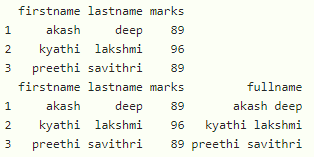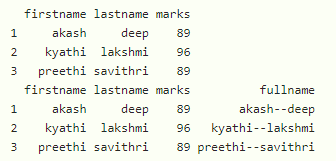Open in App
Not now

# How to Combine Two Columns into One in R dataframe?

• Last Updated : 04 Dec, 2021

In this article, we will discuss how to combine two columns into one in dataframe in R Programming Language.

## Method 1 : Using paste() function

This function is used to join the two columns in the dataframe with a separator.

Syntax:

`paste(data\$column1, data\$column2, sep=" ")`

where

• data is the input dataframe
• column1 is the first column
• column2 to is the second column
• sep is the separator to be separated between two columns

Example 1:

## R

 `# create dataframe``data = ``data.frame``(firstname=``c``(``"akash"``, ``"kyathi"``, ``"preethi"``),``                  ``lastname=``c``(``"deep"``, ``"lakshmi"``, ``"savithri"``),``                  ``marks=``c``(89, 96, 89))` `# display``print``(data)` `# combine first name and last name columns``# with blank separator``data\$fullname = ``paste``(data\$firstname, data\$lastname, sep=``" "``)` `# display``data`

Output:Example 2:

## R

 `# create dataframe``data = ``data.frame``(firstname=``c``(``"akash"``, ``"kyathi"``, ``"preethi"``),``                  ``lastname=``c``(``"deep"``, ``"lakshmi"``, ``"savithri"``),``                  ``marks=``c``(89, 96, 89))` `# display``print``(data)` `# combine first name and last name columns``# with blank separator``data\$fullname = ``paste``(data\$firstname, data\$lastname, sep=``"--"``)` `# display``data`

Output:## Method 2: Using unite() function

This function is available in tidyr package and we have to load that package and will combine columns.

Syntax:

`unite(dataframe, combined_columnname, c(columns))`

where,

• dataframe is the input dataframe
• columns are the dataframe columns to be combines
• combined_columnname is the name of the combined column

Example:

## R

 `# import tidyr package``library``(tidyr)` `# create dataframe``data = ``data.frame``(firstname=``c``(``"akash"``, ``"kyathi"``, ``"preethi"``),``                  ``lastname=``c``(``"deep"``, ``"lakshmi"``, ``"savithri"``),``                  ``marks=``c``(89, 96, 89))` `# display``print``(data)` `# combine first name and last name columns``# with blank separator``data = ``unite``(data, fullname, ``c``(firstname, lastname))` `# display``data`

Output:My Personal Notes arrow_drop_up